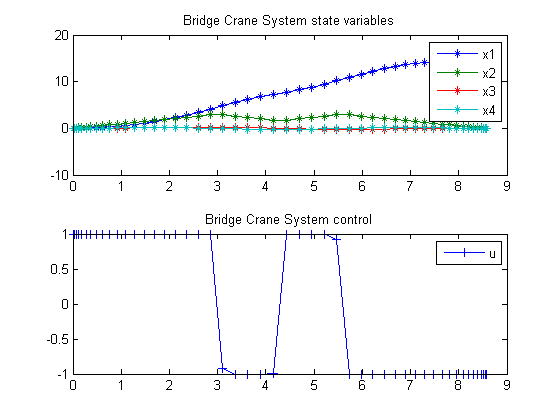16  Bridge Crane System

ITERATIVE DYNAMIC PROGRAMMING, REIN LUUS

12.4.1 Example 1: Bridge crane system

CHAPMAN & HALL/CRC Monographs and Surveys in Pure and Applied Mathematics

16.1  Problem description

Find u over t in [0; t_F ] to minimize

 J = tF

subject to:

 dx1 dt
= x2
 dx2 dt
= u
 dx3 dt
= x4
 dx4 dt
= −0.98*x3 + 0.1*u

The initial condition are:

 x(0)  = [0  0  0  0]
 x(tF) = [15  0  0  0]
 −1 <= u <= 1

Reference: 

16.2  Problem setup

toms t
toms t_f

p = tomPhase('p', t, 0, t_f, 50);
setPhase(p);

tomStates x1 x2 x3 x4
tomControls u

% Initial guess
% Note: The guess for t_f must appear in the list before expression involving t.
x0 = {t_f == 8, ...
collocate(u==1-2*t/t_f)};

% Box constraints
cbox = {0.1 <= t_f <= 100
-1  <= collocate(u) <= 1};

% Boundary constraints
cbnd = {initial({x1 == 0; x2 == 0
x3 == 0; x4 == 0})
final({x1 == 15; x2 == 0
x3 == 0; x4 == 0})};

% ODEs and path constraints
ceq = collocate({
dot(x1) == x2
dot(x2) == u
dot(x3) == x4
dot(x4) == -0.98*x3+0.1*u});

% Objective
objective = t_f;

16.3  Solve the problem

options = struct;
options.name = 'Bridge Crane System';
solution = ezsolve(objective, {cbox, cbnd, ceq}, x0, options);
t  = subs(collocate(t),solution);
x1 = subs(collocate(x1),solution);
x2 = subs(collocate(x2),solution);
x3 = subs(collocate(x3),solution);
x4 = subs(collocate(x4),solution);
u  = subs(collocate(u),solution);
Problem type appears to be: lpcon
Starting numeric solver
===== * * * =================================================================== * * *
TOMLAB - Tomlab Optimization Inc. Development license  999001. Valid to 2011-02-05
=====================================================================================
Problem: ---  1: Bridge Crane System            f_k       8.578933610367178300
sum(|constr|)      0.000000187955007808
f(x_k) + sum(|constr|)      8.578933798322186300
f(x_0)      8.000000000000000000

Solver: snopt.  EXIT=0.  INFORM=1.
SNOPT 7.2-5 NLP code
Optimality conditions satisfied

FuncEv    1 ConstrEv   37 ConJacEv   37 Iter   19 MinorIter  501
CPU time: 0.468750 sec. Elapsed time: 0.469000 sec.

16.4  Plot result

subplot(2,1,1)
plot(t,x1,'*-',t,x2,'*-',t,x3,'*-',t,x4,'*-');
legend('x1','x2','x3','x4');
title('Bridge Crane System state variables');

subplot(2,1,2)
plot(t,u,'+-');
legend('u');
title('Bridge Crane System control');